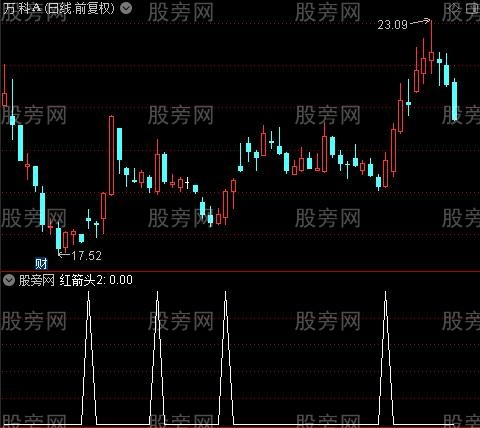KDJ超牛预判之红箭头选股指标公式此处内容已被隐藏，请输入验证码查看内容。

N:=9;
P1:=3;
P2:=3;
RSV:=(CLOSE-LLV(LOW,N))/(HHV(HIGH,N)-LLV(LOW,N))*100;
K:=SMA(RSV,P1,1),COLORCYAN;
D:=Sma(K,P2,1),COLOR00DD00;相关文章